# Chemical reactions and equations class 10 ncert solutions & intext solutions

## Chemical reactions and equations class 10 Intext solutions

Page 6
Question 1
Why should a magnesium ribbon be cleaned before burning in air?
Solution When magnesium ribbon is stored, it reacts with oxygen to form a layer of magnesium oxide. This layer of magnesium oxide is quite stable and prevents further reaction of magnesium with oxygen. Hence a magnesium ribbon should be cleaned before burning in air.

Question 2
Write the balanced equation for the following reaction:
(a) Hydrogen +Chlorine =Hydrogen chloride
(b) Barium chloride+Aluminium sulphate = Barium sulphate +Aluminium chloride
(c) Sodium +Water = Sodium hydroxide + Hydrogen
Solution:
How to solve these type of Question
• First convert the word equation into skeletal equation
• Start by finding out how many atoms of each type are on each side of the equation
• You can make  a little table listing the numbers of each atom for the left hand side and for the right hand side
• Balance the different Atoms on both side of equation by  multiply the chemical species on the side which does not have enough atoms of that type by the number required to bring it up to the same as the other side.
(a)H2 +Cl2     -> 2HCL
(b) 3BaCl2+Al2(SO4) -> 3BaSO4 + 2AlCl3
(c) 2Na + 2H2O  -> 2NaOH + H2

Question 3
Write a balanced chemical equation with state symbols for the following reaction:
(a) Solution of barium chloride and sodium sulphate in water react to give insoluble barium sulphate and the solution of sodium chloride.
(b) Sodium hydroxide solution ( in water ) reacts with hydrochloric acid solution (in water) to produce sodium chloride solution and water.
Solution:
How to solve these type of Question
• First convert the word equation into skeletal equation
• Start by finding out how many atoms of each type are on each side of the equation
• You can make  a little table listing the numbers of each atom for the left hand side and for the right hand side
• Balance the different Atoms on both side of equation by  multiply the chemical species on the side which does not have enough atoms of that type by the number required to bring it up to the same as the other side.
(a) BaCl2(aq) +Na2SO4(aq)-> BaSO 4(s) +2NaCl(aq)
(b) NaOH(aq) +HCl-> NaCl(aq) +H2 O

Page 10
Question 4
A solution of a substance “X” is used for white washing.
(a) Name the substances “x” and write its formula.
(b) Write reaction of the substance “X” named in (!) above with water.
Solution:
(a) The substance “x’ is calcium oxide . Its chemical formula is CaO.
(b) Calcium oxide reacts vigorously with water to form calcium hydroxide ( slaked lime)
CaO+ H2O-> Ca(OH)2

Question 5
Why is the amount of gas collected in one of the test tubes in activity 1.7 double of the amount collected in the other? Name this gas.
Solution:
Water (H2O)contains two parts hydrogen and one part oxygen . Therefore, the amount of hydrogen and oxygen produce during electrolysis of water is in a 2:1 ratio. During electrolysis, since hydrogen goes to one test tube and oxygen goes to another, the amount of gas collected in of the test tubes is double of the amount collected in the other. The gas collected in double the amount is hydrogen and the other gas is oxygen.

Page 13
Question 6
Why does the colour of copper sulphate solution change when an iron nail is dipped in it.
Solution: When an iron nail is placed in a copper sulphate solution , iron displaces copper from copper sulphate solution forming ferrous sulphate solution and copper metal. Ferrous sulphate is green in colour and copper metal is brown.
Therefore, the blue colour of copper sulphate fades and green colour appears.

Question 7
Give an example of a double displacement reaction other than the one given in Activity 1.10.
Solution
A double displacement reaction between the reactants lead nitrate and potassium iodide gives new substances lead iodide and potassium nitrate.
Pb(NO3 )2(aq) +KL(aq)-> PbL2(s) +KNO3(aq)
In this reaction lead nitrate and potassium iodide exchange ion to form two new compounds  lead iodide and potassium nitrate. Hence , it is a double  placement reaction.
Question 8
Identified the substances that are oxidized and the substances that are reduced in the following reaction.
(a) 4Na(s)+ O2(g) -> 2Na2O(s)
(b) CuO(s) + H2(g)-> Cu(s) +H2O(I)
Solution
(a) Sodium (Na) is oxidized to sodium oxide as it gains oxygen and oxygen get reduced.
(b)Copper oxide (CuO) is reduced to copper (Cu) while hydrogen (H2) gets oxidized to water (H2O).

## NCERT Solutions Chemical reactions and equations

Question 9
Which of the statement about the reaction below are incorrect?
2PbO(s) +C(s) -> 2Pb(s) +CO2(g)
2. Carbon dioxide is getting oxidized.
3. Carbon is getting oxidized.
4. Lead oxide is getting reduced
(i)(a) and (b)
(ii) (a) and (c)
(iii) (a) (b) and (c)
(iv) all
Solution
Oxidation is gain of oxygen and reduction reaction is released of oxygen
(a) and (b)

Question 10
Fe2O3 + 2Al -> AL2O3+ 2Fe
The above reaction is an example of
1. Combination reaction
2. Double displacement reaction
3. Decomposition reaction
4. Displacement reaction
Solution
(d) displacement reaction

Question 11
What happens when dilute hydrochloric acid is added to iron fillings? Tick the correct Solution.
(a)Hydrogen gas and iron chloride are produced.
(b) Chlorine gas and iron hydrochloric are produced.
(c) No reaction takes place.
(d) Iron salt and water are produced.
Solution
(a) Hydrogen gas and iron chloride are produced.

Question 12
What is a balance chemical equation? Why chemical equation should is balanced?
Solution
A chemical equation is balanced when the number of atoms of each type  involved in a chemical reaction are same on both the reactant and product sides of the equation.
According to the law of conservation of mass, when a chemical reaction occurs, the mass of the products should be equal to the mass of the reactants. Therefore, the amount of the atoms in each element does not change in the chemical reaction. As a result, the chemical equation that shows the chemical reaction needs to be balanced. A balanced chemical equation occurs when the number of the atoms involved in the reactants side is equal to the number

Question 13
Translate the following statements into chemical equations and then balance them.
(a)Hydrogen gas combines with nitrogen to form ammonia
(b) Hydrogen sulphide gas burns in air to give water and sulphur dioxide.
(c) Barium chloride reacts with aluminium sulphate to give aluminium chloride and a precipitate of barium sulphate
(d) Potassium metal reacts with water to give potassium hydroxide and hydrogen gas.
Solution:
How to solve these type of Question
• First convert the word equation into skeletal equation
• Start by finding out how many atoms of each type are on each side of the equation
• You can make a little table listing the numbers of each atom for the left hand side and for the right hand side
• Balance the different Atoms on both side of equation by  multiply the chemical species on the side which doesn't have enough atoms of that type by the number required to bring it up to the same as the other side.
(a) 3H2 +N2-> 2NH3
(b) 2H2S + 3O2 -> 2H2O + 2SO2
(c) 3BaCl2 + Al2(SO4)3 -> 2AlCl3 + 3BaSO4
(d) 2K + 2H2O -> 2KOH + H2

Question 14
Balance the following chemical equation.
(a)HNO3 + Ca(OH)2-> Ca(NO3)2 +H2O
(b) NaOH + H2SO4 ->Na2SO4+H2O
(c) NaCl + AgNO3 -> AgCl + NaNO3
(d) BaCl2 + H2SO4 -> BaSO4 + HCl
Solution:
How to solve these type of Question
• First convert the word equation into skeletal equation
• Start by finding out how many atoms of each type are on each side of the equation
• You can make  a little table listing the numbers of each atom for the left hand side and for the right hand side
• Balance the different Atoms on both side of equation by  multiply the chemical species on the side which doesn't have enough atoms of that type by the number required to bring it up to the same as the other side.
(a) 2HNO3 + Ca(OH)2 -> Ca(NO3)2 + 2H2O
(b) 2NaOH + H2SO4 -> Na2SO4 + 2H 2O
(c) NaCl + AgNO3-> AgCl + NaNO3
(d) BaCl2 + H2SO 4 -> BaSO4 + 2HCl

Question 15
Write the balance chemical equation for the following reaction.
(a) Calcium hydroxide +Carbon dioxide -> Calcium carbonate + Water
(b) Zinc + Silver Nitrate -> Zinc Nitrate + Silver
(c) Aluminium +Copper chloride -> Aluminium Chloride + Copper
(d) Barium chloride + Potassium sulphate -> Barium Sulphate + Potassium chloride.
Solution:
How to solve these type of Question
• First convert the word equation into skeletal equation
• Start by finding out how many atoms of each type are on each side of the equation
• You can make a little table listing the numbers of each atom for the left hand side and for the right hand side
• Balance the different Atoms on both side of equation by  multiply the chemical species on the side which doesn't have enough atoms of that type by the number required to bring it up to the same as the other side.
(a) Ca(OH)2+ CO2-> CaCO3+H2O
(b) Zn + 2AgNO3 -> Zn(NO3)3 + 2Ag
(c) 2Al + 3CuCl2 -> 2AlCl3 + 3Cu
(d) BaCl2 +K2SO4 -> BaSO4 +2KCl

Question 16
Write the balance chemical equation for the following and identify the type of reaction in each case.
(a) Potassium bromide (aq) + Barium iodide(aq) -> Potassium iodide(aq) + Barium bromide(s)
(b) Zinc carbonate(s) -> Zinc oxide(s) + Carbon dioxide(g)
(c) Hydrogen (g) + chlorine(g) -> Hydrogen chloride(g)
(d) Magnesium (s) + Hydrochloric acid (aq) -> Magnesium chloride(aq) +Hydrogen (g)
Solution:
(a) 2KBr(aq) + BaI2 -> 2KI(aq) + BaBr2(s) Double displacement reaction.
(b) ZnCO3(s)-> ZnO(s) + CO2 Decomposition reaction.
(c) H2(g) +Cl2 (g) -> 2HCl(g) Combination reaction.
(d) Mg(s) + 2HCl(ag) -> MgCl 2(s) + H 2(g) Displacement reaction

Question 17
What does one mean by exothermic and endothermic reaction? Give examples.
Solution: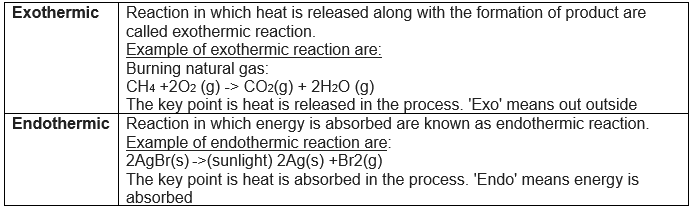Question 18
Why is respiration considered an exothermic reaction ? explain
Solution:
The steps to respiration are
Step 1 :Food that we eat includes carbohydrates, proteins etc.
Step 2: During digestion , carbohydrates are broken down in simpler substances called glucose.
Step 3: Glucose combines with oxygen in the cells of our body to provide energy.
This reaction is called respiration. Since energy is releases during this process, respiration is an exothermic reaction.
C6H 12O 6 (aq) + 6O 2(g) -> 6CO 2(aq) +6H 2O(i) + Energy

Question 19
Why are decomposition reactions called the opposite of combination reaction ? Write equation for these  reactions.
Solution: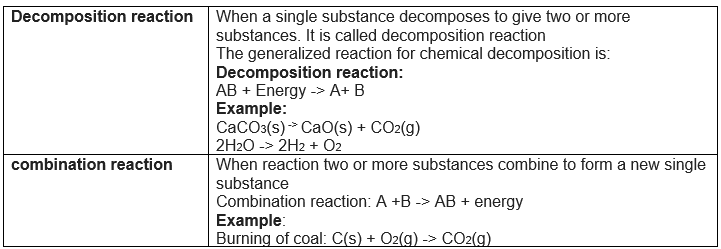So it is clear that Decomposition reaction are opposite of combination reactions.

Question 20
Write one equation each for decomposition reaction where energy is supplied in the form of heat , light or electricity.
Solution:
We already know that When a single substance decomposes to give two or more substances .It is called decomposition reaction
So examples are
 Heat CaCO3(s) -> CaO(s) + CO2(g) Light 2AgBr(s)-> 2Ag(s) + Br2(g) Electricity 2H2O(I) <-> 2H 2 + O2(g)

Question 21
What is the difference between displacement and double displacement reaction ? Write equation for these reactions.
Solution: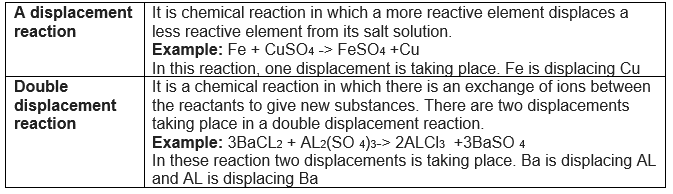Question 22
In refining of silver, the recovery of silver from silver nitrate solution involved displacement by copper metal. Write down the reactions involved
Solution:
2AgNO3(aq) + Cu(s)-> Cu(NO 3)2 + 2Ag(s)
Question 23:
What do mean by precipitation reaction? Explain by giving example.
Solution:
Important points are
(1)Any reaction that produces a precipitate can be called a precipitation reaction.
(2) Precipitation reaction produce insoluble salts which settle down as precipitate.
For example : When aqueous sodium sulphate solution and aqueous barium chloride are reacted, aqueous solution of sodium chloride and white precipitate of barium sulphate are formed.
Na2SO4(aq) + BaCL2(aq) -> BaSO4(s) + 2NaCL(aq)

Question 23
Explain the following in terms of gain or loss of oxygen with two examples each.
(a) Oxidation
(b) Reduction
Solution: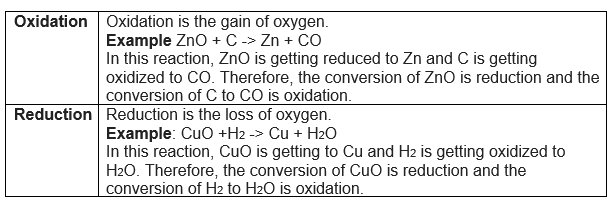Question 24
A shiny brown coloured element “X” on heating in air becomes black in colour. Name the element “X” and the black coloured compound formed.
Solution:
The element “X” is copper and the black coloured compound is copper oxide (CuO). The chemical reaction is :
2Cu + O2 -> 2CuO

Question 25
Why we do apply paint on iron articles?
Solution:
Iron react with air and moisture and corrode .So we apply paint on iron articles to avoid rusting of iron. Paint will stop the contact of air and moisture with iron.

Question 26
Oil and fat containing food items are  flushed with nitrogen. Why?
Solution:
When fats and oil are oxidized , they become rancid and their smell and taste change. Food items containing fat and oil are flushed with nitrogen to prevent rancidity of oil and fat as nitrogen is an inert gas and prevent the oxidation of oil and fats.

Question 27
Explain the following terms with one example each.
(a) Corrosion
(b) Rancidity
Solution: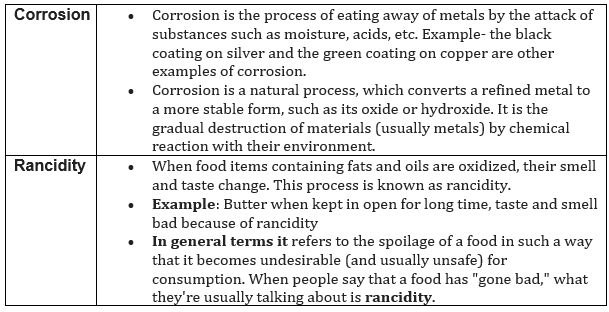## Summary

1. NCERT (intext and Exercise ) Solution Class 10 Chapter 1 Chemical reactions and equations has been prepared by Expert with utmost care. If you find any mistake.Please do provide feedback on mail. You can download the solutions as PDF in the below Link also